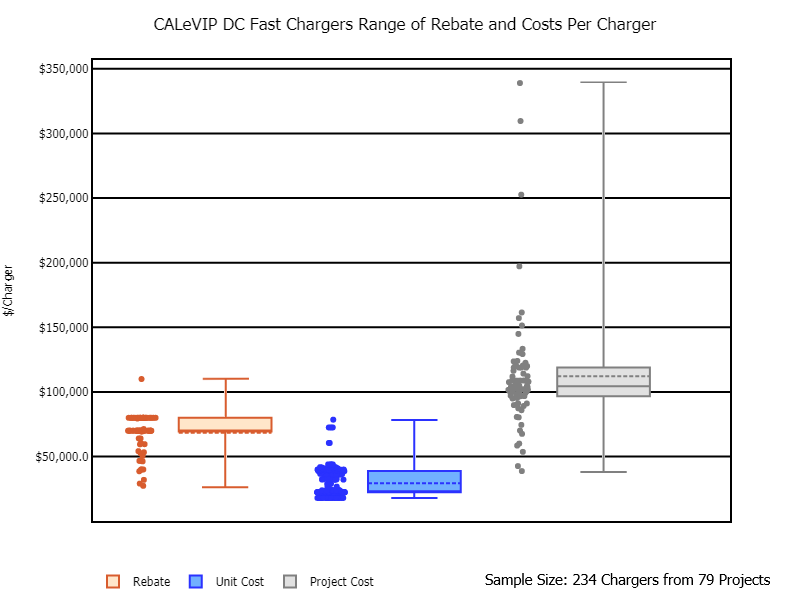The box plots show the range of rebate per charger, unit cost per charger, and total project cost per charger for direct current (DC) fast charger CALeVIP projects. The rebate per charger ranged from a minimum of \$27,374 to a maximum of \$110,000 with a mean and median of \$68,413 and \$70,000, respectively. The unit cost per charger ranged from a minimum of \$18,000 to a maximum of \$78,540 with a mean and median of \$29,406 and \$23,000, respectively. The total project cost per charger ranged from a minimum of \$38,793 to a maximum of \$338,938 with a mean and median of \$112,099 and \$104,326, respectively.

Each point shown next to the respective box plot represents the rebate, unit cost, and total project cost for one project. The top and bottom ends of the box are the upper and lower quartiles, respectively. The box spans the interquartile range. The horizontal solid line within the box marks the median value. The horizontal dotted line within the box represents the mean value. The whiskers are the two lines that extend above and below the box and represent the highest and lowest values. This graph excludes about half of DC fast charger sites where the unit cost was undefined because of aggregated equipment costs. This graph excludes sites where the site host installed both Level 2 and DC Fast Chargers under a single rebate.

## Contact

Fuels and Transportation Division
FTD@energy.ca.gov